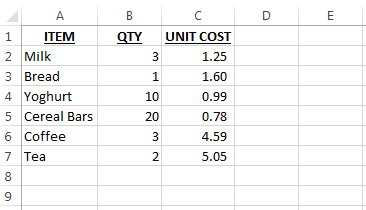# SUMPRODUCTContinuing the series of posts about useful, but sometimes underused Excel formulas, this post will give you an introduction to SUMPRODUCT.

SUMPRODUCT is one formula that pretty much combines two together.

• SUM : When you add two or more numbers together, the result of these is the “SUM” of them.
• PRODUCT : When you multiply two or more numbers together, this gives you the “PRODUCT”.

The SUMPRODUCT formula gives you the product of a given array and returns the sum of those products.

## SHOPPING LIST

To demonstrate and explain the SUMPRODUCT formula, we will use the example of a shopping list.We have 6 items on our list, a quantity to buy and a unit cost for each item.

## PRODUCT

One way to get the total value of our shopping would be to multiply the quantity by the unit cost to get the total cost for each individual item.  This is probably how you are used to seeing something like this done.The values in column D are the product of the values in columns B and C.

## SUM

By adding all the values in column D, we get the total value of our shopping list.  This is the sum of the products of our quantities and unit costs.Note the sum of the products is 54.72.   We will check this against our SUMPRODUCT in the next step.

## SUMPRODUCT

The syntax for the SUMPRODUCT formula is =sumproduct(array1, [array2],[array3]…).

An array is a systematic arrangement of objects.  In our case, the list of quantities is one array and the list of unit costs is another array.

Why are our lists arrays?  The lists are organised so the first quantity is matched to the first unit cost, the second quantity is matched to the second unit cost and so on.

The SUMPRODUCT formula will get the product of the first items in each array, then the second in each array, then the third in each array and so on until it has gone through the arrays.  Then it gets the sum of all these products.

The range of quantites in our file is from B2 down to B7.  This is our first array.

The range of unit costs in our file is from C2 down to C7.  This is our first array.

We only have two arrays in our example, but SUMPRODUCT allows for many more arrays.

Our formula is =SUMPRODUCT(B2:B7, C2:C7).

As explained above, this gets the product of each item in array 1 and 2, and then sums those products.Note that the result of our SUMPRODUCT is the same as we worked out above when we got totalled the product of each individual item.

Plus, you don’t have to type in your ranges for the arrays, you can highlight the cells with your mouse/touchpad.

## WHY USE SUMPRODUCT?

You are probably already comfortable with the straightforward sum formula and multiplying 2 numbers together – so why not get the product and the sum of the products?

That works, I used to do this and hide the column/rows that contained the multiplication formulas and summed them.

Later, I came across some templates that used SUMPRODUCT formula to work out priority numbers for lists of items to be investigated.  Since then, I have been using the SUMPRODUCT formula – it works, as does the old way of using 2 formulas.

You may find that with Excel, there is usually more than one way to get the same result.